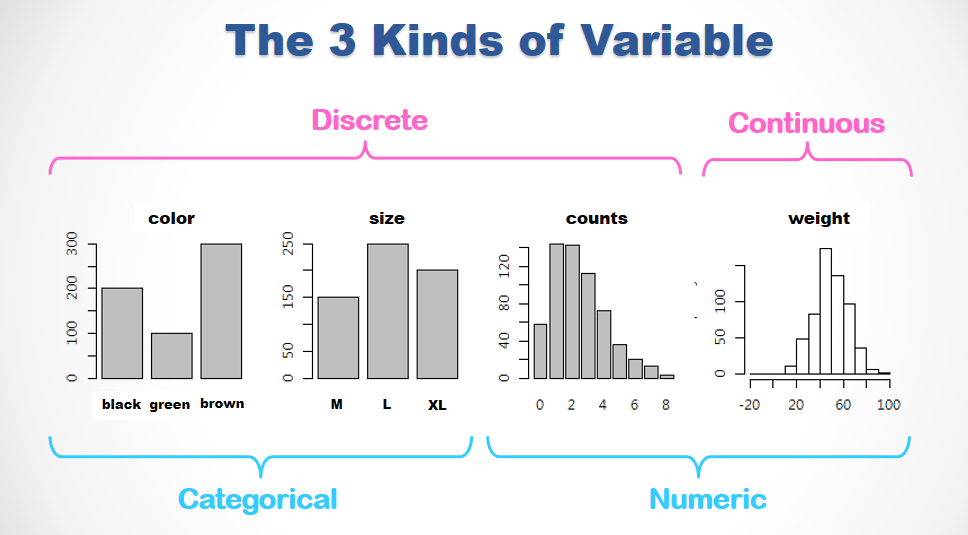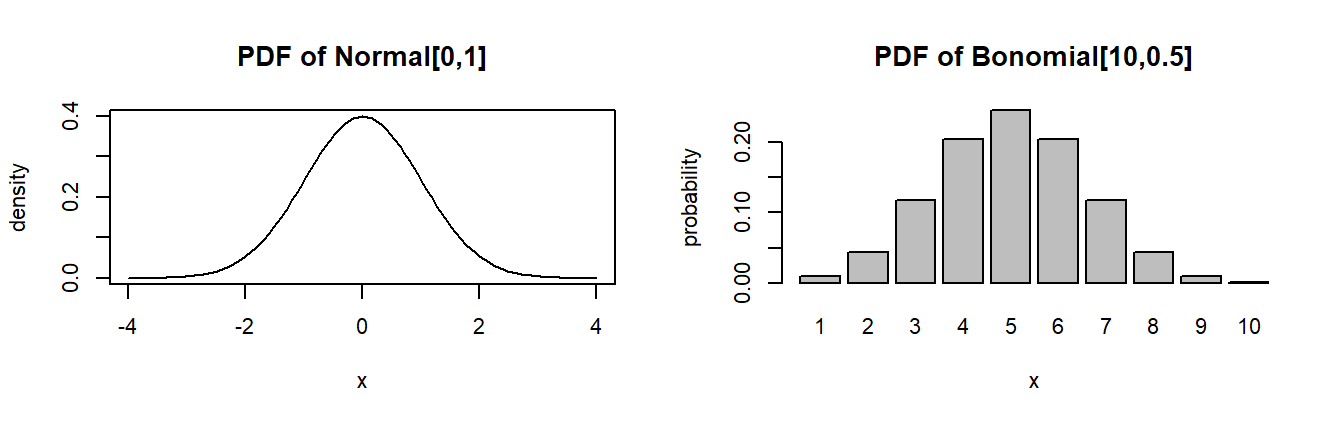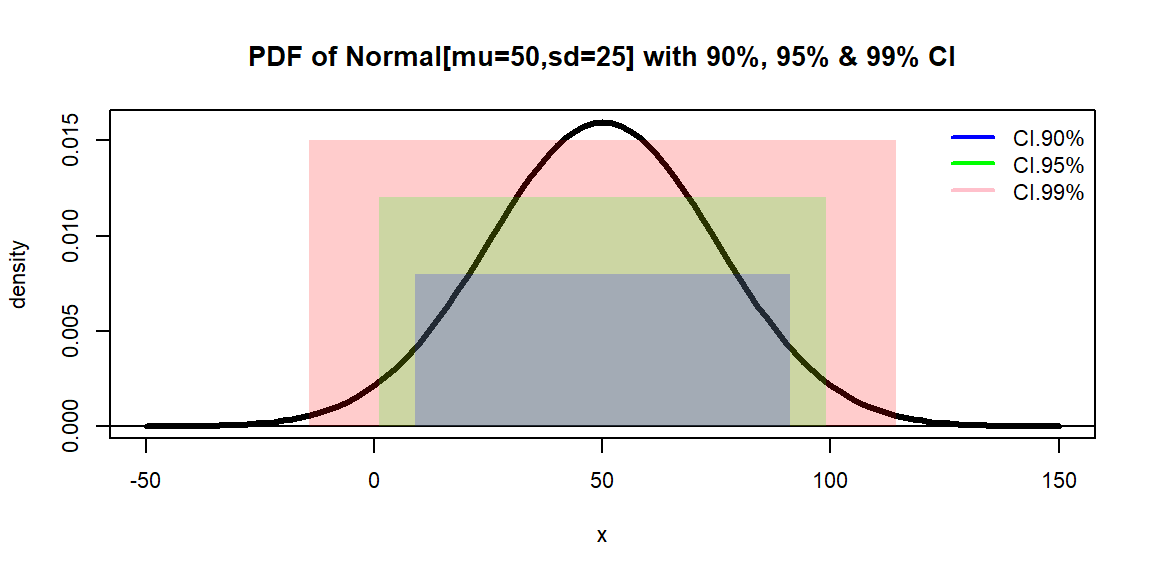👨‍🏫 KEY POINTS :

🔑 Distribution: the connections among probability, probability density and probability density function (PDF/CDF).

🔑 3 different types of variables. Each has a distinctive distribution form.

🔑 A same set of operations generally apply to all kinds of distribution.

🔑 Put distribution functions in use.

🔑 Define random variables by simulating random experiment

pacman::p_load(dplyr, vcd, future.apply)
plan(multisession)

### 1. The 3 types of Variable/Distribution

There are 3 types of variables. Categorical variables are always discrete. Their distributions are represented by counts and barplots. Numerical variables are either Discrete (integers) or Continuous (real numbers). The former are visualized by barplots and the latter histograms. The distributions of both Numeric types may be precisely specified by mathematical formula named after PDF and CDF.##### 1.1 Built-in Distribution Functions

Most of the commonly used distributions functions are built-in in R (See the online help of distributions for details.)

• beta : dbeta()
• binomial : dbinom()
• Cauchy : dcauchy()
• chi-squared : dchisq()
• exponential : dexp()
• F : df()
• gamma : dgamma()
• geometric : dgeom
• hypergeometric : dhyper()
• log-normal : dlnorm()
• multinomial : dmultinom()
• negative binomial : dnbinom()
• normal : dnorm()
• Poisson : dpois()
• Student’s t : dt()
• uniform : dunif()
• Weibull : dweibull()

and there are more of them in various packages.

##### 1.2 The 4 Operations upon Distibutions

There are 4 common operations that generally apply to every distributions.

• d<dist>(x, <args>) : for probability density function (PDF)
• p<dist>(q, <args>) : for cumulative distribution function (CDF)
• q<dist>(p, <args>) : for quantile function, the reversed CDF
• r<dist>(n, <args>) : for random sampling

where <dist> specifies a specific distribution and <args> represent the corresponding arguments. For examples

• _norm(., mean, sd) for Normal[mean,sd] and
• _binom(., size, prob) for Binomial[size,prob].

These distribution operation function will be elaborated in the following sections.

### 2. Prob. Density Function (PDF) - d<dist>

For a continuous distribution such as dnorm(x, mean, sd) returns the probability density at x for Normal[mean,sd].

For a discrete distribution such as dbinom(x, size, prob) returns the probability at x for Binomial[size,prob].

Usually the d functions are used to plot PDF.

par(mfrow=c(1,2), cex=0.7)
curve(dnorm(x), -4, 4,
main="PDF of Normal[0,1]", ylab="density")
barplot(dbinom(1:10,10,0.5),names=1:10,
main="PDF of Bonomial[10,0.5]", ylab="probability", xlab="x")🦋 DISCUSSION :

Try to elaborate the difference between the continuous and discrete distributions in the following aspects …

🐞 the mathematical forms of PDF

🐞 the plotting codes

🐞 the label on the y-axis

🐞 What is probability density? How is it different from probability itself?

🚴 Exercise :

👉 Acquire the prob. densitity of Standard Normal Dist. (Normal[0,1]) at -3:3

#
#

👉 Acquire and Plot the prob. densities of Normal[100,20] at seq(50,120,10). Plot with type='h',lwd=5,col='pink and label the plot with the appropriate main, xlab, ylab arguments in plot().

#
#

👉 Acquire the probabilities of Binomial[10,1/6] at c(0,2,4,6,8,10)

#
#

👉 Roll a fair dice 10 times, what is the probability of having exactly 2 one’s.

#
#

👉 Roll a fair dice 20 times, what is the probability of having less than 5 one’s.

#
#

### 3. Cumulative Density Function (CDF) - p<dist>

For any continuous distributions, there is no probability at any given point. Therefore we usually define each event as a specific range of value. If we were to acquire the probability of such an event with PDF, we’d have to do calculus integration over the range. By integrating PDF from its left tail, CDF offers a easier way to acquire the probability over a range. For an example, the probability of $$x \in [-1,2]$$ in Standard Normal Dist. can be acquired by subtracting the CDF reading at the upper bound to that at the lower.

#
#

🚴 Exercise :

👉 Acquire the prob. of Standard Normal Dist. in the interval [-Inf, -3].

#
#

👉 Acquire the prob. of Normal[100,50] in the interval [0, 50].

#
#

👉 If the distribution of the temperature at this season follows Normal[25,10] Celsius, what is the probability that tomorrow’s average temperature is higher than 30°C.

#
#

👉 Flipping a fair coin 100 times, what is the probability to have less than 35 heads?

#
#

👉 Can you validate your answer of the above question by using PDF?

#
#

👉 Rolling a fair dice 100 times. What is the probability to have no greater than 20 and no less than 10 one’s?

#
#

👉 Try to validate your answer of the above question by using PDF.

#
#

See? For discrete distributions, PDF serves most of the purposes most of the time.

🚴 SIMULATION :

Let’s also try to estimate the above probability by sample() and replicate().

n = replicate(20000, sum(sample(1:6, 100, T) == 1))
a = mean(n>=10 & n<=20)
c(a, a - sum(dbinom(10:20,100,1/6)))
  0.8243000 -0.0025197

👉 Wrap the above code chunk into another replicate(), repeat 10 times and observe

• How long does it takes?
• What are the average and the largest error?
#
#

What have we learned from the previous exercise?
When we have a theoretical distribution with known arguments, acquiring the probability directly from the corresponding PDF/CDF is easier, faster and more accurate than doing simulation.

Nonetheless, our real world is full with cases that are not covered in any of the pre-defined distributions. For an example, if we wanted to estimate the probability that every side of the dice occurs no greater than 20 and no less than 10 …

K = 20000
n = replicate(K, sample(factor(1:6), 100, T) %>% table %>% range)
mean(n[1,]>=10 &  n[2,]<=20)
 0.261

Run the above code chuck a few times. You’d see that the event occurs in about 25.8% of the K=20000 trials. To assure the comvergence, we can wrap the above code chuck into future_replicate() which replicates in parallel.

t0 = Sys.time()
a = future_replicate(24, {
n = replicate(K, sample(factor(1:6), 100, T) %>% table %>% range)
mean(n[1,]>=10 &  n[2,]<=20)
}, future.seed=TRUE)
c(mean(a), sd(a))
 0.2598583 0.0031781
Sys.time() - t0
Time difference of 13.41 secs

In the 24 replications, the mean and standard deviation of the estimated probability is 0.2596 and 0.0035 respectively. The small standard deviation indicates that the trial size of K=20000 is large enough for convergence.

### 4. Random Sampling - r<dist>

r<dist>(n, <args>) randomly draws n data points from the <dist> of known <args>. For examples …

norm50 = rnorm(n=50, mean=50, sd=10)  # draw a sample of size 50 from Normal[50,10]
unif50 = runif(n=50, min=0, max=100)  # draw a 50-point sample from Uniform[50,10]  

These random sample functions are very useful in business simulation. Supposed we know the profit contribution of a customer $$i$$ - $$\pi_i$$

$\pi_i(V_i, X_i) = \left\{\begin{matrix} \sqrt{X_i} & , & V_i <= 2\\ \sqrt{X_i+20} & , & V_i \in [3,4]\\ 20+\sqrt{X_i} & , & V_i >= 5\\ \end{matrix}\right.$

is determined by two random variables:

• the number of store visit per month $$V \sim Poison[\lambda=3.25]$$ and
• the transaction amount $$X \sim Normal[\mu=180, \sigma=15]$$

It is difficult to determine the distribution of $$\pi$$ with math. Let’s try to do this via simulation

Pi.Trial = function(K) {
pi = data.frame(
V = rpois(K, lambda=3.25),
X = rnorm(K, mean=180, sd= 15)
) %>%
mutate(
X = pmax(0, X),
pi = case_when(
V <= 2 ~ sqrt(X),
V >= 5 ~ 20+sqrt(X),
TRUE ~ sqrt(X+20)
) ) %>% pull(pi)
c(mu=mean(pi), sigma=sd(pi))
}
Pi.Trial(1e5)
     mu   sigma
18.2901  8.2809 

See? Within a second, we can create a sample 0f 100K (1e5) and estimate the mu and sigma for the distribution of pi.

To see the precision of our estimation we can replicate the trial

K = 1e5
future_replicate(24, Pi.Trial(K), future.seed=T) %>%
t %>% as.data.frame %>%
summarise_all(.funs=list(mean=mean, sd=sd))
  mu_mean sigma_mean    mu_sd sigma_sd
1  18.252     8.2527 0.032373 0.020578

🚴 Exercise :

According to the Central Limit Theories, standard deviation is reversely proportional to the square root of the sample size. So …

🐞 If the sd’s are to reduce by a half, the sample size should be multiplied by …?

👉 Modify the above code chuck to reduce the sd of our estimations in half.

#
#

🐞 Is the Central Limit Theories still working in the 21st century?

### 5. Quantile Functions - q<dist>

The q<dist> functions are the reverse p<dist> functions. For a random variable X ~ <dist>[<args>], q<dist>(p, <args>) returns the critical value q whereof Prob[x <= q] = p.

The q<dist> functions is widely used in the statistical method of hypothesis testing. Assuming X ~ Normal[mu, sigma], qnorm(c(0.025, 0.975), mu, sigma) returns the critical values x1 and x2 whereof Prob[ X ∈ [x1, x2] ] = 0.975 - 0.025 = 0.95. Because the probability that X falls in [x1, x2] is 95% , this interval is called 95% confidence interval in Statistic textbooks.

qnorm(c(0.025, 0.975), 100, 20)
  60.801 139.199

🚴 Exercise :

With X ~ Normal[mu=50, sigma=25]

👉 Acquire the 99% and 95% and 90% confidence intervals of X.

 -14.3957   1.0009   8.8787  91.1213  98.9991 114.3957`

👉 Plot the PDF of X and mark these interval in different colors🌞 TAKE AWAYS :

R is an integrated working environment where we can …

🌻 Draw sample data from known distribution

🌻 Generate sample data by simulating experiment and

🌻 Replicate the experiment to create distribution

🌻 Fit data into theoretic distribution or

🌻 build empirical distribution directly form data

🌻 Operate theoretical, empirical or simulated distributions in the same set of tools.